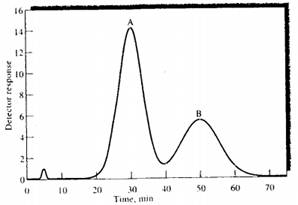### Create an Account

Already have account?

### Forgot Your Password ?

Home / Questions / A chromatogram of a two component mixture on a 25 cm packed LC column is shown in the figu...

# A chromatogram of a two component mixture on a 25 cm packed LC column is shown in the figure below The flow rate was

A chromatogram of a two-component mixture on a 25-cm packed LC column is shown in the figure below. The flow rate was (0.40 mL/min.

(a) Find the times that components A and B spend in the stationary phase.

(b) Find the retention times for A and B.

(c) Determine the retention factors for the two components.

(d) Find the full widths of each peak and the full width at half-maximum values.

(e) Find the resolution of the two peaks.(f) Find the average number of plates for the column.

(g) Find the average plate height.

(h) What column length would be needed to achieve a resolution of 1.75?

(i) What time would be required to achieve the resolution in part (h)?

(j) Assume that the column Length is fixed at 25 cm and the packing material is fixed. What measures could you take to increase the resolution to achieve baseline separation?

(k) Are there any measures you could use to achieve a better separation in a shorter time with the same column as in part (j)?

Jun 23 2020 View more View Less

#### Answer (Solved)Subscribe To Get Solution